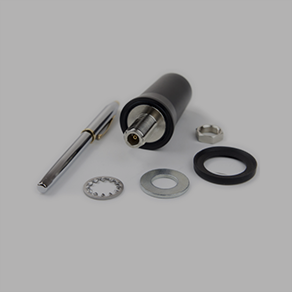Need Help Selecting an Antenna? Contact Us. Free Shipping for all orders over \$50.

# Antenna Ground Plane CalculationQ) I would like to know the difference between RSGB-698/2700-3-NF and TRA6927M3PBN-001, that makes the gains so much different. For the first one, peak gain at 698-960 is 3.5dB and the second one is 0dB.

A) The only difference between the two is that the TRA6927M3PBN-001 does not require a metallic ground plane.  That being said, that would be the answer to the gain-loss as well, as you will lose a substantial amount of gain when the antenna is not mounted on a metal base.

The amount/size of the “ground-plane” required for optimal performance of a monopole antenna is determined by the frequency you are transmitting/receiving at. In this case, it’s a multi-band antenna the customer is using (it can be used from 700 MHz – 2700 MHz) so the ground plane required could be anywhere from 4-64 square inches.

At 700 Mhz: 8x8 inches

At 2700 Mhz: 2x2 inches

You can calculate this by using the free Ground Plane Antenna Calculator tool at http://www.csgnetwork.com/antennagpcalc.html. Simply type the frequency into the field labeled “Desired Frequency” and click “Calculate” The minimum ground plane size required for optimal performance will be displayed below as “radial length”For example, if the ideal radial length is calculated at 4 inches, that means that the ground plane must extend out at least 4” in each direction from the antenna in the center, for a ground plane size of 8x8 inches (64 square inches.) Keep in mind that the ground plane does NOT need to be perfectly shaped, the antenna simply needs to be in contact with a total of 64 square inches of metallic surface area.